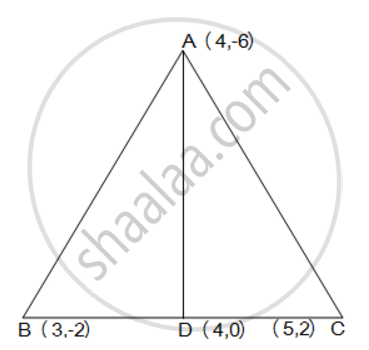Share

# Median of a Triangle Divides It into Two Triangles of Equal Areas. Verify this Result for δAbc Whose Vertices Are a (4, - 6), B (3, - 2) and C (5, 2). - CBSE Class 10 - Mathematics

#### Question

median of a triangle divides it into two triangles of equal areas. Verify this result for ΔABC whose vertices are A (4, - 6), B (3, - 2) and C (5, 2).

#### SolutionLet the vertices of the triangle be A (4, -6), B (3, -2), and C (5, 2).

Let D be the mid-point of side BC of ΔABC. Therefore, AD is the median in ΔABC.

Coordinates of point D = ((3+5)/2, (-2+2)/2) = (4,0)

Area of a triangle =1/2 {x_1 (y_2 - y_3) + x_2 (y_3 - y_1) + x_3 (y_1 - y_2)}

Area of ΔABD =  1/2 [(4) {(-2) - (0)} + 3{(0) - (-6)} + (4) {(-6) - (-2)}]

= 1/2 (-8+18-16)

= -3 square units

However, area cannot be negative. Therefore, area of ΔABD is 3 square units.

Area of ΔABD = 1/2 [(4) {0 - (2)} + 4{(2) - (-6)} + (5) {(-6) - (0)}]

= 1/2 (-8+32-30)

= -3 square units

However, area cannot be negative. Therefore, area of ΔABD is 3 square units.

The area of both sides is same. Thus, median AD has divided ΔABC in two triangles of equal areas

Is there an error in this question or solution?

#### APPEARS IN

NCERT Solution for Mathematics Textbook for Class 10 (2019 to Current)
Chapter 7: Coordinate Geometry
Ex. 7.30 | Q: 5 | Page no. 170

#### Video TutorialsVIEW ALL 

Solution Median of a Triangle Divides It into Two Triangles of Equal Areas. Verify this Result for δAbc Whose Vertices Are a (4, - 6), B (3, - 2) and C (5, 2). Concept: Area of a Triangle.
S